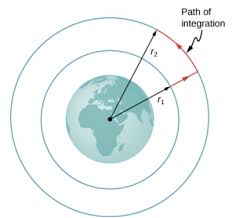## How to Calculate and Solve for Gravitational Potential | The Calculator EncyclopediaThe image above represents the Gravitational potential.

To compute the gravitational potential of a field, two essential parameters are needed which are mass (m) and radius (r).

The formula for calculating the gravitational potential;

V = (Gm) / r

Where;
V = Gravitational potential
m = Mass

Let’s solve an example;
Find the gravitational potential of a field when the mass is 14 cm with a radius of 9 cm.

This implies that;
m = Mass = 14 cm
r = Radius = 9 cm

V = (Gm) / r
V = (6.67 x 10-11 x 14) / 9
V = 1.0375e-10 / 9
V = 1.0375e-10

Therefore, the gravitational potential is 1.0375e-10 Volts (V).